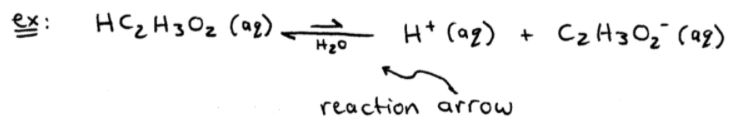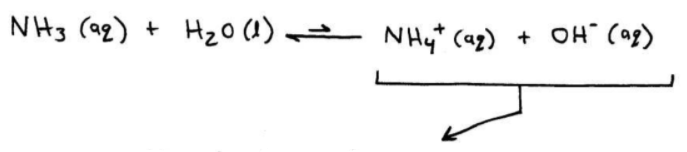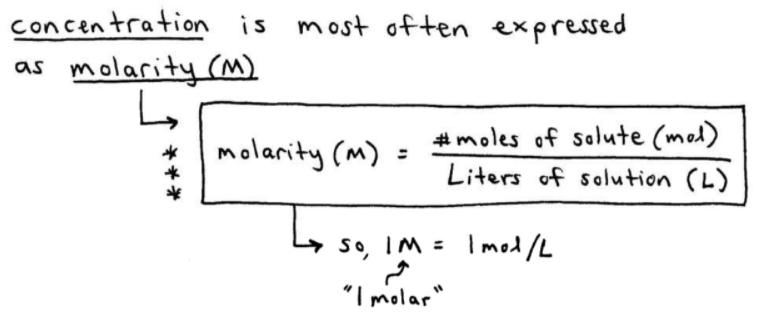# S4E2 - Strong and Weak Electrolytes, Nonelectrolytes, and Molarity

At the end of my first post on SECTION 4 - Types of Chemical Reactions and Solution Stoichiometry, we introduced Strong Electrolytes, Weak Electrolytes, and Nonelectrolytes.

Let's elaborate on each of these 3 types of substances...

## 1. Strong Electrolytes.

Strong Electrolytes = substances (solutes) that are completely ionized (hydrated) when dissolved in water.

ex:  very soluble salts (i.e. NaCl)
ex:  strong acids (i.e. HCl, HNO3, HClO4, H2SO4)
ex:  strong bases (i.e. NaOH, KOH)

➞ when the above compounds are dissolved in water, they are 100% ionized. In other words, they ionize (dissociate into cation and anion) completely:So, the easier it is for a substance (solute) to form ions in solution, the better it is at conducting electricity because ions act as charge carriers in aqueous solutions.

=====

## 2. Weak Electrolytes.

Weak Electrolytes = substances that ionize (dissolve) only slightly in water.

➞ two most common are:  NH3 (ammonia) and HC2H3O2 (acetic acid).

➞ they produce relatively few ions (charge carriers) when dissolved...See the reaction arrow above?  It shows us that mainly only HC2H3O2 is present and it's not an ion.

Because acetic acid (HC2H3O2) is a weak electrolyte, it's called a weak acid.

weak acid = any acid that dissociates (ionizes) only to a slight extent in aqueous solution.

-----

ex:  The most common weak base is ammonia (NH3). It's a weak electrolyte:The solution is basic because OH- ions are produced. But because very few OH- ions are formed, NH3 is a weak electrolyte, and thus a weak base.

=====

## 3. Nonelectrolytes.

Nonelectrolytes = substances that do dissolve in water to make an aqueous solution, but do not produce any ions.

ex:  C2H5OH (ethanol)
ex:  C12H22O11 (sucrose) -- table sugar. Here's what happens:=====

## Molarity and Concentration in Chemistry.

The extent to which a solute dissolves in a solution is expressed by the solution's concentration.

Concentration is most often expressed as molarity (M).

Molarity (M)  =  number of moles of solute (mol)  /  liters of solution (L).

So, 1M  =  1 mol/L.

Here it is again, but easier to follow:## Molarity Calculations.

ex:  Calculate the molarity of a solution made by dissolving 11.5g of solid NaOH in enough water to make 1.50L of solution.

- well, we know that molarity = moles of NaOH / liters of solution.

- we already have the "liters of solution" = 1.50L

- so the first thing we need to do, is calculate the "moles of NaOH"...=====

In my next video blog post from this series: SECTION 4 - Types of Chemical Reactions and Solution Stoichiometry, we'll cover more Molarity Calculations.